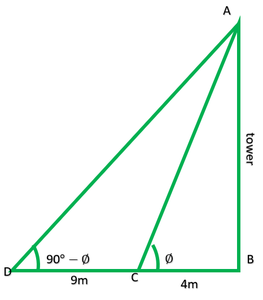Open In App
Related Articles
• NCERT Solutions for Class 10 Maths

# Class 10 NCERT Solutions- Chapter 9 Some Application of Trigonometry – Exercise 9.1 | Set 2

### Question 11. A TV tower stands vertically on a bank of a canal. From a point on the other bank directly opposite the tower, the angle of elevation of the top of the tower is 60°. From another point 20 m away from this point on the line joining this point to the foot of the tower, the angle of elevation of the top of the tower is 30° (see Fig.). Find the height of the tower and the width of the canal.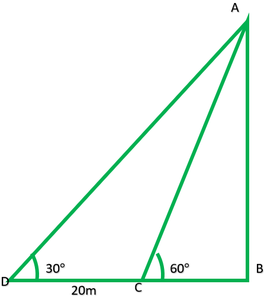Solution:

In fig: AB = tower = ?

CB = canal = ?

In rt. ∆ABC,

tan60° =h/x = √3

h = √3 x          -(1)

In rt. ∆ABD= tan 30°= 1/√3

h = (x + 20)/√3          -(2)

From (1) and (2)

√3/1 = (x + 20)/√3

3x = x + 20

3x – x = 20

2x = 20

X = 20/2

X = 10

Width of the canal is 10m

Putting value of x in equation 1

h = √3 x

= 1.732(10)

= 17.32

Height of the tower 17.32m.

### Question 12. From the top of a 7 m high building, the angle of elevation of the top of a cable tower is 60° and the angle of depression of its foot is 45°. Determine the height of the tower.

Solution:

In fig: ED = building = 7m

AC = cable tower = ?

In rt ∆EDC,= tan45°

7/x = 1/1

DC = 7

Now, EB = DC = 7m

In rt. ∆ABE,= tan60°

AB/7 = √3/1

Height of tower = AC = AB + BC

7√3 + 7

= 7(√3 + 1)

= 7(1.732 + 1)

= 7(2.732)

Height of cable tower = 19.125m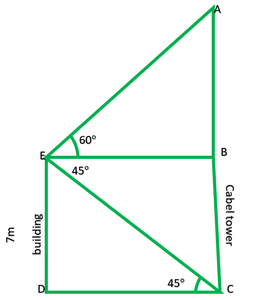### Question 13. As observed from the top of a 75 m high lighthouse from the sea-level, the angles of depression of two ships are 30° and 45°. If one ship is exactly behind the other on the same side of the lighthouse, find the distance between the two ships.

Solution:

In fig:

AB = lighthouse = 75m

D and C are two ships

DC = ?

In rt. ∆ABD,= tan30°

75/BD = 1/√3

BD = 75√3

In rt. ∆ABC= tan45°

75/BC = 1/1

BC = 75

DC = BD – BC

= 75√3 – 75

75(√3 – 1)

75(1.372 – 1)

34.900

Hence, distance between two sheep is 34.900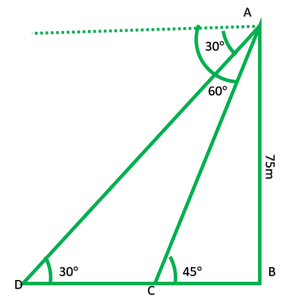### Question 14. A 1.2 m tall girl spots a balloon moving with the wind in a horizontal line at a height of 88.2 m from the ground. The height of 88.2 m from the ground. The angle of elevation of the balloon from the eyes of the girl at any instant is 60°. After some time, the angle of elevation reduces to 30° (see Fig.). Find the distance traveled by the balloon during the interval.

Solution:

In fig: AB = AC – BC

= 88.2 – 1.2

= 81m

In rt. ∆ABE= 87/EB = tan30°

87/EB = 1/√3

EB = 87√3

In rt. ∆FDE= tan60°

√3 ED = 87

ED = 87/√3

DB = DB – ED

87√3 – 87/√3

87(√3 – 1/√3)

= 87(3 – 1/√3)

= 87(2/√3) = 174/√3 * √3/√3

= 174 * √3/3 = 58√3

58 * 1.732 = 100.456m

Distance traveled by balloon is 100.456m

### Question 15. A straight highway leads to the foot of a tower. A man standing at the top of the tower observes a car at an angle of depression of 30°, which is approaching the foot of the tower with a uniform speed. Six seconds later, the angle of depression of the car is found to be 60°. Find the time taken by the car to reach the foot of the tower from this point.

Solution:

In fig: AB is tower

In rt. ∆ABD= tan30°= 1/√3

DB = √3 AB          -(1)

In rt. ∆ABC= tan60°

BC = AB/√3          -(2)

DC = DB – BC

= √3 AB – AB/√3

AB(3 – 1/√3)

CD = 2AB/√3S1 = S2

\frac{D1}{T1} = \frac{D2}{T2}2/√3AB/6 = AB/√3/t

2t = 6

t = 6/2

t = 3sec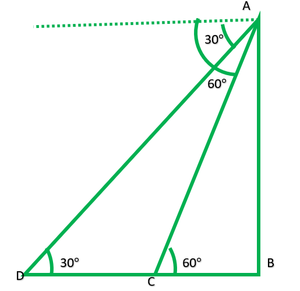### Question 16. The angles of elevation of the top of a tower from two points at a distance of 4 m and 9 m from the base of the tower and in the same straight line with it are complementary. Prove that the height of the tower is 6 m.

Solution:

In fig: AB is tower

To prove: AB = 6m

Given: BC = 4m          DB = 9m

In ∆ABC= tanθ

AB/4 = tanθ         -(1)

In ∆ABD= tan (90°-θ)

AB/9 = 1/ tanθ

9/AB = tanθ         -(2)

From (1) and (2)

AB/4 = 9/AB

AB2 = 36

AB = √36

AB = √(6 * 6)

AB = 6m

Height of the tower is 6m.